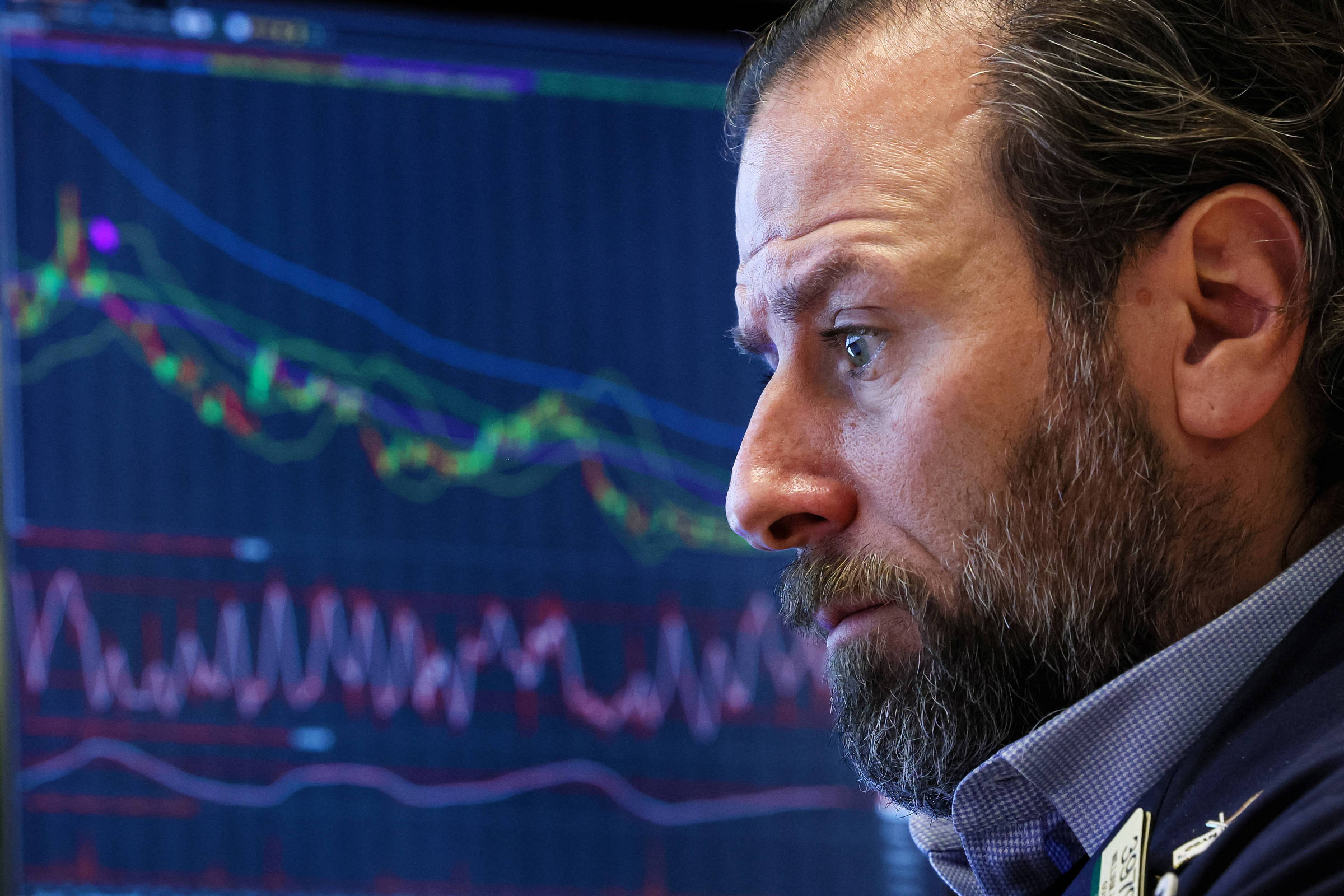# Understand why volatility is an important measure of risk and how to interpret it – 11/19/2023 – From Grão to GrãoHave you ever heard a manager say that the volatility of his fund is around 4% per year or that the stock market volume is 22.7% per year? Usually called vol, volatility is a measure widely used to express the risk of a financial asset, but little understood by investors. I will explain how you can interpret it in a practical way.

First let’s understand how it is calculated. However, I will try to explain it intuitively, that is, without formulas. For reasons of understanding, I will try to be very simplistic. But for those who want to calculate, vol is simply the standard deviation of returns.

I will explain through an example. Let’s use the monthly returns of Ibovespa.

In the last 240 months, that is, in 20 years, if we calculate the average monthly returns of the Ibovespa we will find 1%. However, each month there was a different return. In the best month, Ibovespa returned 17% and in the worst -29.9%. However, the average of these returns gave 1% return per month.

Volatility would be a kind of average of the differences of each monthly return observation in relation to this 1% return. Translated, you are calculating how much on average an asset’s returns differ from its average return.

Active Vol. a year Vol. per month
IPCA Treasury (IMAB-5) 3.1% 0.9%
Multimarket Funds 4.0% 1.2%
7-10 year American Treasury 6.5% 1.9%
IPCA Treasury (IMAB-5+) 10.6% 3.1%
Real Estate Funds 10.9% 3.1%
American Treasury +20 years 13.5% 3.9%
S&P500 14.8% 4.3%
Dollar 15.7% 4.5%
Gold 16.9% 4.9%
Nasdaq 17.8% 5.1%
Commodities 19.0% 5.5%
Imob Fund USA 22.1% 6.4%
Ibovespa 22.7% 6.6%

The table above expresses the annual and monthly volatility of various assets. Annual data are usually calculated from monthly data, multiplying them by root 12. I won’t go into the details of why this happens, but it is simply the way the standard deviation is calculated.

It is possible to observe that Ibovespa is the asset with the highest volume, that is, we know that it is the one that presents the greatest risk. However, how do you interpret this vol information?

To interpret the concept of volatility it is necessary to assume a probability distribution for returns. It is usually assumed that returns follow a Normal distribution. This is the famous bell-shaped distribution shown in the figure below.

This same figure highlights how you should interpret volatility information. Notice that there is a mark in the center of the graph. This represents the average return on the asset.

From this central measurement, there are marks on the right side that are separated by the acronyms +1s, +2s and +3s. The letter s represents the volatility measure and the number a multiplier. The same occurs on the left side, but with a negative sign.

Note that there are three numbers below the graph identifying a type of distance. In fact, it is the probability that a random observation is between the marks.

It is this probability that we must use to interpret volatility.

For example, we know that the average monthly return of Ibovespa is 1% and its monthly volume is 6.6%. This means that with a 68.26% probability, the Ibovespa return next month could be between -5.5% (=1% – 1*6.6%) and +7.6% (=1% + 1 *6.6%).

Looking at it another way, there is a 34.13% probability that next month’s return will be between 1% and 7.6% and a 34.13% probability that next month’s return will be between -5.5% and 1%

Also, with a 95.44% probability, the Ibovespa return next month could be between -12.2% (=1% – 2*6.6%) and +14.2% (=1% + 2* 6.6%).

It seems like a really big range, doesn’t it? But this is what you should expect from assets with higher vol, that is, higher risk. You are less sure about what will happen.

Therefore, the volatility measure offers you sensitivity to the uncertainty about an asset’s future returns. The lower the volume of an asset, the closer its future return tends to be to its average return.

For example, IMAB-5’s average monthly return is also 1% over the last 20 years and its monthly volatility is just 0.9%. Therefore, monthly return results tend to be closer to the same 1% than in the case of Ibovespa.

This risk measure is important so that you understand the magnitude of unfavorable results that may occur and their probability. This way, you can better define whether a certain investment is for you, as you would be able to know which unfavorable results you will have to endure.

Michael Viriato is an investment advisor and founding partner of Investor’s House.

Speak directly to me via email.

Follow and like De Grão em Grão on social media. Follow investment lessons on Instagram.

LINK PRESENT: Did you like this text? Subscribers can access five free accesses from any link per day. Just click the blue F below.